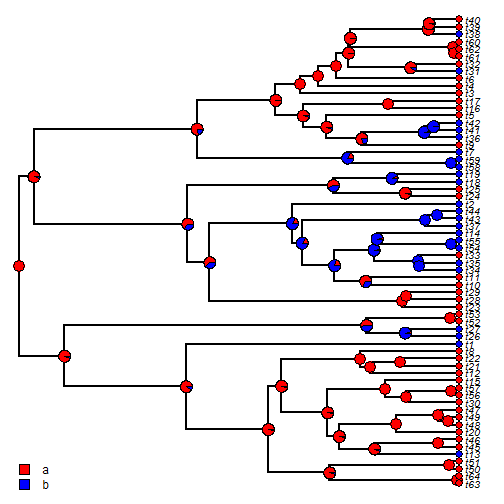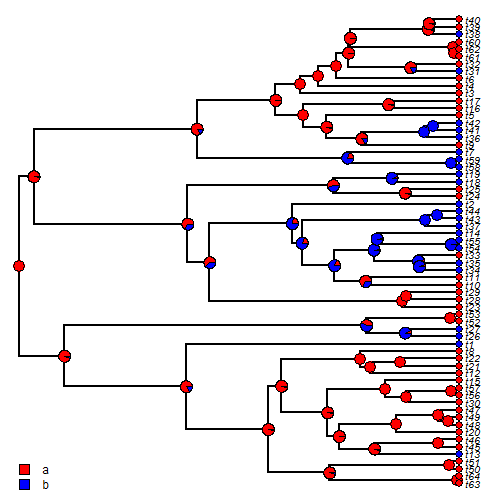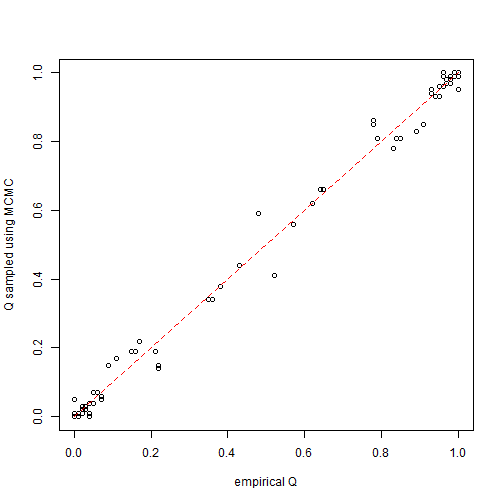## Saturday, March 11, 2017

### Bug fixes for non-flat prior on the root node in `make.simmap` (and for MCMC method)

A couple of different phytools users have reported a bug (e.g., here) in `make.simmap` for values of the prior distribution on the root node other than the default.

This bug was one that I evidently accidentally introduced into `make.simmap` with the best intention of allowing users to send optional arguments to the internally used function `fitMk` for optimizing the transition model or computing the likelihood.

I believe that I have now fixed this bug; however I await user reports to the contrary (should they be necessary).

I also detected and (hopefully) fixed (1, 2) a second bug in `make.simmap(...,Q="mcmc")` that I believe I must have introduced at the same time.

Just to mention a little bit about the difference between the default method (`Q="empirical"`) and `Q="mcmc"`. The default method first maximizes the likelihood of the transition matrix, Q and then uses this “empirical” value of Q to sample character histories for the discrete trait from their posterior distribution. By contrast, `Q="mcmc"` is essentially a fully heirarchical Bayesian method in which (first) `nsim` values of Q are sampled from their posterior probability distribution using Bayesian MCMC. and then (next) `nsim` character histories are obtained via stochastic mapping. In the event that multiple trees are input, an MCMC will be run on each tree.

Here's a quick demo on simulated data. (Simulation conditions are given at the end.

First, let's try the `Q="empirical"` (default) method; however I will also impose a prior on the root node to have state `"a"` with probability `0.90`:

``````library(phytools)
packageVersion("phytools")
``````
``````##  '0.5.81'
``````
``````tree
``````
``````##
## Phylogenetic tree with 64 tips and 63 internal nodes.
##
## Tip labels:
##  t63, t64, t50, t51, t13, t45, ...
##
## Rooted; includes branch lengths.
``````
``````x
``````
``````## t63 t64 t50 t51 t13 t45 t46 t20 t48 t49 t47 t30 t56 t57 t15 t12 t21 t22
## "a" "a" "a" "a" "b" "a" "a" "a" "a" "a" "a" "a" "a" "a" "a" "a" "a" "a"
##  t8  t1 t26 t27 t52 t53 t23 t28 t29 t10 t11 t34 t35 t33 t54 t55 t14 t37
## "a" "b" "b" "b" "a" "a" "a" "a" "a" "a" "a" "b" "b" "a" "b" "b" "b" "b"
## t43 t44  t2 t24 t25 t18 t19 t58 t59  t7  t9 t36 t41 t42  t5 t16 t17  t3
## "b" "b" "b" "a" "a" "b" "b" "b" "b" "b" "a" "b" "b" "b" "a" "a" "a" "a"
##  t4  t6 t31 t32 t61 t62 t60 t38 t39 t40
## "a" "a" "b" "a" "a" "a" "a" "b" "a" "a"
``````
``````## prior on root node
pi<-setNames(c(0.9,0.1),c("a","b"))
pi
``````
``````##   a   b
## 0.9 0.1
``````
``````empirical<-make.simmap(tree,x,nsim=100,pi=pi,Q="empirical")
``````
``````## make.simmap is sampling character histories conditioned on the transition matrix
##
## Q =
##           a         b
## a -1.136101  1.136101
## b  1.136101 -1.136101
## (estimated using likelihood);
## and (mean) root node prior probabilities
## pi =
##   a   b
## 0.9 0.1
``````
``````## Done.
``````
``````sum.emp<-summary(empirical)
sum.emp
``````
``````## 100 trees with a mapped discrete character with states:
##  a, b
##
## trees have 18.31 changes between states on average
##
## changes are of the following types:
##        a,b  b,a
## x->y 11.54 6.77
##
## mean total time spent in each state is:
##               a         b    total
## raw  11.0705364 4.9707401 16.04128
## prop  0.6901281 0.3098719  1.00000
``````
``````cols<-setNames(c("red","blue"),c("a","b"))
plot(sum.emp,colors=cols,fsize=0.8,ftype="i")
``````Now we can compare the `Q="mcmc"` method as follows:

``````mcmc<-make.simmap(tree,x,nsim=100,pi=pi,Q="mcmc")
``````
``````## Running MCMC burn-in. Please wait....
## Running 10000 generations of MCMC, sampling every 100 generations.
##
## make.simmap is simulating with a sample of Q from
## the posterior distribution
##
## Mean Q from the posterior is
## Q =
##           a         b
## a -1.135897  1.135897
## b  1.135897 -1.135897
## and (mean) root node prior probabilities
## pi =
##   a   b
## 0.9 0.1
``````
``````## Done.
``````
``````sum.mcmc<-summary(mcmc)
sum.mcmc
``````
``````## 100 trees with a mapped discrete character with states:
##  a, b
##
## trees have 18.49 changes between states on average
##
## changes are of the following types:
##        a,b  b,a
## x->y 11.73 6.76
##
## mean total time spent in each state is:
##               a         b    total
## raw  11.0470643 4.9942122 16.04128
## prop  0.6886649 0.3113351  1.00000
``````
``````cols<-setNames(c("red","blue"),c("a","b"))
plot(sum.mcmc,colors=cols,fsize=0.8,ftype="i")
``````Most likely, at least under the defaults for both methods, our posterior probabilities at all nodes should be similar:

``````plot(sum.emp\$ace,sum.mcmc\$ace,xlab="empirical Q",ylab="Q sampled using MCMC")
lines(c(0,1),c(0,1),lty="dashed",col="red")
``````The tree & data for this demo were simulated as follows:

``````Q<-matrix(c(-1,1,1,-1),2,2,dimnames=list(letters[1:2],letters[1:2]))
x<-getStates(sim.history(tree<-pbtree(n=64,scale=1),Q,
anc="a"),"tips")
``````

1.Nice! This will solve the issue. Thanks!

2.Hi,

When trying to set Q to "estimated" I get the following message: formal argument "Q" matched by multiple actual arguments

LIkewise, when trying to set a pi at the root, I get the message: formal argument "pi" matched by multiple actual arguments.

Please, would anyone know the reason why?

1.This is a bug that I believe should already be fixed. Which phytools version are you using? If the latest CRAN version, and you still encounter this problem, I would recommend installing from GitHub using devtools.

Note: due to the very large amount of spam, all comments are now automatically submitted for moderation.